﻿ a尺度二元最小能量多小波框架的构造

# a尺度二元最小能量多小波框架的构造The Construction of Bivariate Minimum-Energy Multi-Wavelet Frames with Dilation Factor a

Abstract: Based on the minimum-energy multi-wavelet frames, the concept of bivariate minimum-energy multi-wavelet frames with dilation factor a and the sufficient and necessary conditions for satisfying the characteristics of bivariate minimum energy multi-wavelet frames are given. Via using the polyphase decomposition that corresponds to the symbol functions for scaling function and wavelet function, the decomposition and reconstruction algorithms of bivariate minimum-energy multi-wavelet frames with dilation factor a are presented.

1. 引言

2. a尺度二元最小能量多小波框架的概念

$〈f,g〉={\int }_{{R}^{2}}\text{ }f\left(x\right)\stackrel{¯}{g\left(x\right)}\text{ }\text{d}x,\text{\hspace{0.17em}}\forall f,g\in L\left(R2\right)$

$\stackrel{^}{f}\left(\omega \right)={\int }_{{R}^{2}}\text{ }f\left(x\right){\text{e}}^{-i〈x,\omega 〉}\text{d}x$

$A{‖f‖}^{2}\le \underset{i=1}{\overset{N}{\sum }}\underset{\tau =1}{\overset{r}{\sum }}\underset{j,k\in {Z}^{2}}{\sum }{|〈f,{\psi }_{\tau ,j,k}^{i}〉|}^{2}\le B{‖f‖}^{2},\text{\hspace{0.17em}}\forall f\left(x\right)\in {L}^{2}\left(R2\right)$

$‖f\left(x\right)‖=\underset{\tau =1}{\overset{r}{\sum }}\underset{j,k\in {Z}^{2}}{\sum }{|〈f,{\psi }_{\tau ,j,k}^{i}〉|}^{2},\text{\hspace{0.17em}}\forall f\in {L}^{2}\left({R}^{2}\right)$ (1)

${V}_{k}=clo{s}_{{L}^{2}\left\{{R}^{2}\right\}}\left(span\left\{{\varphi }_{k,\alpha }\left(x\right):\alpha \in {Z}^{2}\right\}\right)$$\left\{{\varphi }_{k,\alpha }\left(x\right):\alpha \in {Z}^{2}\right\}$${L}^{2}\left({R}^{2}\right)$ 上的闭包。

(1) $\cdots \subset {V}_{\tau }\subset {V}_{\tau +1}\subset {V}_{\tau +2}\subset \cdots ,\text{\hspace{0.17em}}\tau \in Z$

(2) $\stackrel{¯}{{\cup }_{\tau \in Z}\text{ }{V}_{\tau }}={L}^{2}\left({R}^{2}\right),\text{\hspace{0.17em}}{\cap }_{\tau \in Z}\text{ }{V}_{\tau }=\left\{0\right\}$

(3) $g\left(x\right)\in {V}_{0}⇔g\left(x-k\right)\in {V}_{0},\text{\hspace{0.17em}}k\in {Z}^{2}$

(4) $g\left(x\right)\in {V}_{\tau }⇔g\left(ax\right)\in {V}_{\tau +1},\text{\hspace{0.17em}}\tau \in Z$

(5) $\exists \varphi \left(x\right)\in {L}^{2}\left({R}^{2}\right)$ 使 ${\left\{\varphi \left(x-k\right)\right\}}_{k\in {Z}^{2}}$${V}_{0}$ 的紧框架，那么 ${\left\{{V}_{\tau }\right\}}_{\tau \in Z}$ 是FMRA，其中 $\varphi \left(x\right)$ 是FMRA的二

$\underset{\tau =1}{\overset{r}{\sum }}\underset{k\in {Z}^{2}}{\sum }{|〈f,{\varphi }_{\tau ,1,k}〉|}^{2}=\underset{\tau =1}{\overset{r}{\sum }}\underset{k\in {Z}^{2}}{\sum }{|〈f,{\varphi }_{\tau ,0,k}〉|}^{2}+\underset{i=1}{\overset{N}{\sum }}\underset{\tau =1}{\overset{r}{\sum }}\underset{k\in {Z}^{2}}{\sum }{|〈f,{\psi }_{\tau ,0,k}^{i}〉|}^{2}$ (2)

${\varphi }_{j,k}={a}^{j}\varphi \left({a}^{j}x-k\right),\text{\hspace{0.17em}}{\psi }_{j,k}^{i}={a}^{j}{\psi }^{i}\left({a}^{j}x-k\right),\text{\hspace{0.17em}}i,j\in Z,k\in {Z}^{2}$

$\underset{\tau =1}{\overset{r}{\sum }}\underset{k\in {Z}^{2}}{\sum }〈f,{\varphi }_{\tau ,1,k}〉{\varphi }_{\tau ,1,k}=\underset{\tau =1}{\overset{r}{\sum }}\underset{k\in {Z}^{2}}{\sum }〈f,{\varphi }_{\tau ,0,k}〉{\varphi }_{\tau ,0,k}+\underset{i=1}{\overset{N}{\sum }}\underset{\tau =1}{\overset{r}{\sum }}\underset{k\in {Z}^{2}}{\sum }〈f,{\psi }_{\tau ,0,k}^{i}〉{\psi }_{\tau ,0,k}^{i}$ (3)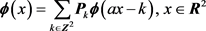(4)(5)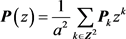(6)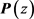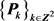的符号函数。对于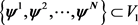根据定义2，就有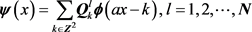(7)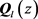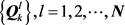的符号函数，表示为(8)(9)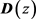的共轭转置我们用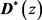来表示。

3. a尺度二元最小能量多小波框架的特征

(1)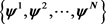为与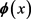相应的二元最小能量多小波框架生成元；

(2)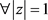满足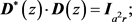(10)

(3)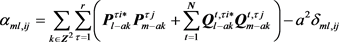(11)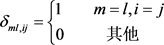(12)(13)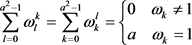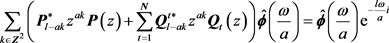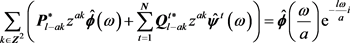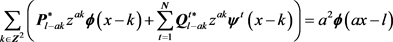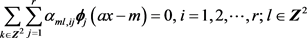(14)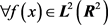是紧支撑函数，若固定m，则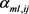仅有有限个不为零，那么泛函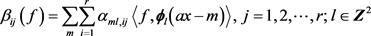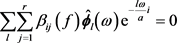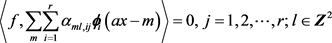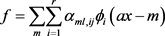，则有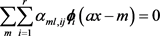，最后做傅里叶变换就可得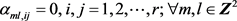，综上，定理得证。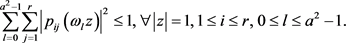(15)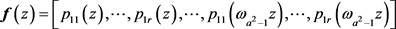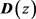的第一行元素，将中剩下的记为矩阵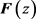，那么根据式(10)就有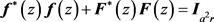是Hermitian矩阵，那么就有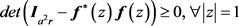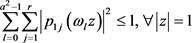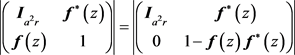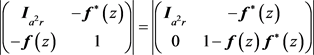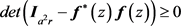所以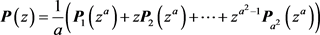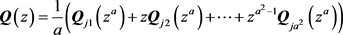阶的符号矩阵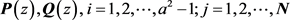中的每项都为Laurent多项式，记为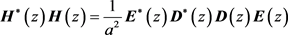(16)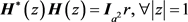(17)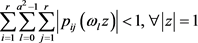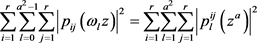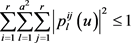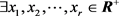且有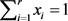，则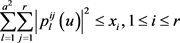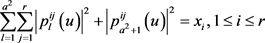4. 二元最小能量多小波框架的构造算法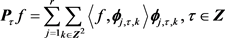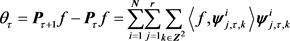(18)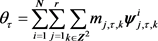(19)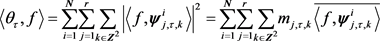1) 分解算法 若已知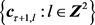，那么我们由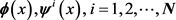的加细方程我们可以得到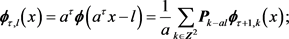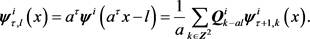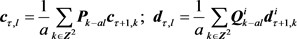2) 重构算法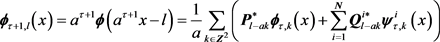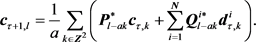Liang, Q. and Zhao, P. (2010) Minimum-Energy Multi-Wavelets Tight Frames Associated with Two Scaling Functions. International Conference on Wavelet Analysis and Pattern Recognition, Qingdao, 11-14 July 2010, 97-101.
https://doi.org/10.1109/ICMLC.2010.5581087

 梁倩. 两个尺度函数生成的最小能量多小波紧框架[D]: [硕士学位论文]. 北京: 北京交通大学, 2010.

 高协平, 曹春红. 最小能量区间小波框架研究[J]. 中国科学:科学技术版, 2009, 39(4): 441-453.

 Chui, C.K. and He, W. (2000) Compactly Supported Tight Frames Associated with Refinable Functions. Applied and Computational Harmonic Analysis, 8, 293-319.
https://doi.org/10.1006/acha.2000.0301

 陈清江, 李婷婷, 雷晓婷. 二元最小能量小波框架的特征刻画[J]. 应用数学, 2017, 30(3): 595-602.

 何永滔. 紧支撑的最小能量框架[J]. 中山大学学报, 2011, 33(2): 166-176.

 陈清江, 李杰. 二元小波紧框架存在的充分条件[J]. 云南大学学报, 2008, 30(3): 217-223.

 王刚. 二元三带小波紧框架的构造[J]. 应用数学学报, 2008, 31(2): 290-305.

 郭蔚, 彭立中. 多小波框架的构造理论[J]. 中国科学, 2010, 40(10): 1115-1128.

 黄永东, 李秋富. a进制最小能量区间小波框架的构造[J]. 中国科学: 信息科学, 2013, 43(4): 469-487.

 Huang, Y.D. and Cheng, Z.X. (2007) Minimum Energy Frames Associated with Refinable Function of Arbitraty Inter Dilation Factor. Chaos, Solitons and Fractals, 32, 503-515.
https://doi.org/10.1016/j.chaos.2006.06.082

Top